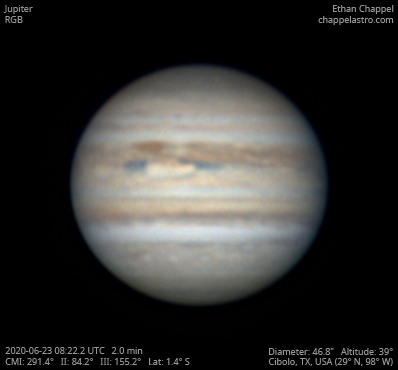# Jupiter 2020-06-23 08:22 UTC

CM1: 291.40°

CM2: 84.20°

CM3: 155.20°

CLat: -1.40°

Description

I had taken in the C8, but the sky cleared again. Instead of risking a repeat of what happened earlier, I pulled out my Sky-Watcher Classic 150P to quickly get some nice views through the eyepiece. However, I decided to see what this telescope is capable of and inserted my ASI224MC. Although thin clouds interfered with the imaging, I can't complain about the results for a setup worth a few hundred dollars.

Equipment

ZWO ASI224MC

Celestron 2x

Logs
```FireCapture v2.6  Settings
------------------------------------
Observer=Ethan Chappel
Location=Cibolo
Scope=Celestron C14 EdgeHD
Camera=ZWO ASI224MC
Filter=None
Profile=Jupiter
Diameter=46.76"
Magnitude=-2.69
CMI=291.6° CMII=84.4° CMIII=155.4°  (during mid of capture)
FocalLength=2600mm (F/7)
Resolution=0.30"
Filename=2020-06-23-0822_7-EC-None-Jup.ser
Date=2020_06_23
Start=08_22_16.756
Mid=08_22_46.760
End=08_23_16.764
Start(UT)=08_22_16.756
Mid(UT)=08_22_46.760
End(UT)=08_23_16.764
Duration=60.008s
Date_format=yyyy_MM_dd
Time_format=HH_mm_ss
LT=UT -6h
Frames captured=2713
File type=SER
Binning=no
ROI=256x244
ROI(Offset)=0x0
FPS (avg.)=45
Shutter=5.000ms
Gain=374 (62%)
FPS=100 (off)
HardwareBin=off
AutoExposure=off
Gamma=50
WRed=52 (off)
AutoHisto=75 (off)
HighSpeed=off
Brightness=1 (off)
SoftwareGain=10 (off)
AutoGain=off
WBlue=95 (off)
USBTraffic=100 (off)
Histogramm(min)=0
Histogramm(max)=181
Histogramm=70%
Noise(avg.deviation)=0.60
Limit=60 Seconds
Sensor temperature=32.6°C
FireCapture v2.6  Settings
------------------------------------
Observer=Ethan Chappel
Location=Cibolo
Scope=Celestron C14 EdgeHD
Camera=ZWO ASI224MC
Filter=None
Profile=Jupiter
Diameter=46.76"
Magnitude=-2.69
CMI=291.0° CMII=83.7° CMIII=154.8°  (during mid of capture)
FocalLength=2600mm (F/7)
Resolution=0.30"
Filename=2020-06-23-0821_7-EC-None-Jup.ser
Date=2020_06_23
Start=08_21_13.534
Mid=08_21_43.540
End=08_22_13.546
Start(UT)=08_21_13.534
Mid(UT)=08_21_43.540
End(UT)=08_22_13.546
Duration=60.012s
Date_format=yyyy_MM_dd
Time_format=HH_mm_ss
LT=UT -6h
Frames captured=2201
File type=SER
Binning=no
ROI=256x244
ROI(Offset)=0x0
FPS (avg.)=36
Shutter=5.000ms
Gain=374 (62%)
FPS=100 (off)
HardwareBin=off
AutoExposure=off
Gamma=50
WRed=52 (off)
AutoHisto=75 (off)
HighSpeed=off
Brightness=1 (off)
SoftwareGain=10 (off)
AutoGain=off
WBlue=95 (off)
USBTraffic=100 (off)
Histogramm(min)=0
Histogramm(max)=183
Histogramm=71%
Noise(avg.deviation)=0.59
Limit=60 Seconds
Sensor temperature=32.5°C
```